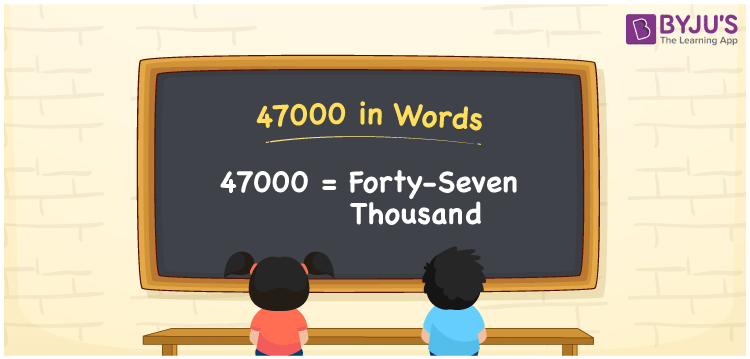# 47000 in Words

In words, 47000 is written as Forty-seven Thousand. The number name of 47000 is frequently written using place values such as ones, tens, hundreds, and so on. Hence, while writing the number 47000 in words, the place value table proves useful. For example, if you spent Rs. 47000 on purchasing a bike, you can write “I spent Rs. Forty-seven thousand on purchasing a bike”. We will discuss how to spell 47000 in English words as well as how to write the numerical name 47000 in this article.

 47000 in Words: Forty-seven Thousand Forty-seven Thousand in Numerical Form: 47000

## 47000 in English Words## How to Write 47000 in Words?

To write the number 47000 in words, find the place value of each digit. A place value chart for the number 47000 is shown below:

 Ten – Thousands Thousands Hundreds Tens Ones 4 7 0 0 0

As a result, the number’s expanded form is expressed as follows:

= 4 × Ten Thousands + 7 × Thousand × 0 × Hundred × 0 × Ten + 0 × One

= 4 × 10000 + 7 × 1000 + 0 × 100 + 0 × 10 + 0 × 1.

= 47000

= Forty-seven thousand

Hence, 47000 in words is Forty-seven thousand.

After 46999 but before 47001, the natural number 47000 appears.

47000 in words – Forty-seven thousand

Is 47000 an odd number? – No

Is 47000 an even number? – Yes

Is 47000 a perfect square number? – No

Is 47000 a perfect cube number? – No

Is 47000 a prime number? – No

Is 47000 a composite number? – Yes

## Frequently Asked Questions on 47000 in Words

Q1

### Write 47000 in words.

47000 in words is Forty-seven thousand.

Q2

### Simplify 47500 – 500, and express in words.

Simplifying 47500 – 500, we get 47000. Hence, 47000 in words is forty-seven thousand.

Q3

### How to write forty-seven thousand in numbers?

Forty-seven thousand in numbers is 47000.